# mne.VolSourceEstimate#

class mne.VolSourceEstimate(data, vertices, tmin, tstep, subject=None, verbose=None)[source]#

Container for volume source estimates.

Parameters:
data`array` of shape (n_dipoles, n_times) | `tuple`, shape (2,)

The data in source space. The data can either be a single array or a tuple with two arrays: “kernel” shape (n_vertices, n_sensors) and “sens_data” shape (n_sensors, n_times). In this case, the source space data corresponds to `np.dot(kernel, sens_data)`.

vertices

The indices of the dipoles in the source space. Should be a single array of shape (n_dipoles,) unless there are subvolumes.

tminscalar

Time point of the first sample in data.

tstepscalar

Time step between successive samples in data.

subject`str`

The FreeSurfer subject name. While not necessary, it is safer to set the subject parameter to avoid analysis errors.

verbose

Control verbosity of the logging output. If `None`, use the default verbosity level. See the logging documentation and `mne.verbose()` for details. Should only be passed as a keyword argument.

`SourceEstimate`

A container for surface source estimates.

`VectorSourceEstimate`

A container for vector surface source estimates.

`VolVectorSourceEstimate`

A container for volume vector source estimates.

`MixedSourceEstimate`

A container for mixed surface + volume source estimates.

Notes

New in v0.9.0.

Attributes:
subject

The subject name.

`times``array` of shape (n_times,)

A timestamp for each sample.

vertices

The indices of the dipoles in the source space. Should be a single array of shape (n_dipoles,) unless there are subvolumes.

`data``array` of shape (n_dipoles, n_times)

Numpy array of source estimate data.

`shape``tuple`

Shape of the data.

Methods

 Add source estimates. Divide source estimates. Multiply source estimates. Negate the source estimate. Subtract source estimates. `apply_baseline`([baseline, verbose]) Baseline correct source estimate data. `as_volume`(src[, dest, mri_resolution, format]) Export volume source estimate as a nifti object. `bin`(width[, tstart, tstop, func]) Return a source estimate object with data summarized over time bins. Return copy of source estimate instance. `crop`([tmin, tmax, include_tmax]) Restrict SourceEstimate to a time interval. `extract_label_time_course`(labels, src[, ...]) Extract label time courses for lists of labels. `get_peak`([tmin, tmax, mode, vert_as_index, ...]) Get location and latency of peak amplitude. `in_label`(label, mri, src, *[, verbose]) Get a source estimate object restricted to a label. Make a summary stc file with mean over time points. `plot`(src[, subject, subjects_dir, mode, ...]) Plot Nutmeg style volumetric source estimates using nilearn. `plot_3d`([subject, surface, hemi, colormap, ...]) Plot SourceEstimate. `resample`(sfreq[, npad, window, n_jobs, verbose]) Resample data. `save`(fname[, ftype, overwrite, verbose]) Save the source estimates to a file. `save_as_volume`(fname, src[, dest, ...]) Save a volume source estimate in a NIfTI file. Take the square root. Make a summary stc file with sum over time points. `time_as_index`(times[, use_rounding]) Convert time to indices. `to_data_frame`([index, scalings, ...]) Export data in tabular structure as a pandas DataFrame. `transform`(func[, idx, tmin, tmax, copy]) Apply linear transform. `transform_data`(func[, idx, tmin_idx, tmax_idx]) Get data after a linear (time) transform has been applied.

__div__(a)[source]#

Divide source estimates.

__mul__(a)[source]#

Multiply source estimates.

__neg__()[source]#

Negate the source estimate.

__sub__(a)[source]#

Subtract source estimates.

apply_baseline(baseline=(None, 0), *, verbose=None)[source]#

Baseline correct source estimate data.

Parameters:
baseline`None` | `tuple` of length 2

The time interval to consider as “baseline” when applying baseline correction. If `None`, do not apply baseline correction. If a tuple `(a, b)`, the interval is between `a` and `b` (in seconds), including the endpoints. If `a` is `None`, the beginning of the data is used; and if `b` is `None`, it is set to the end of the interval. If `(None, None)`, the entire time interval is used.

Note

The baseline `(a, b)` includes both endpoints, i.e. all timepoints `t` such that `a <= t <= b`.

Correction is applied to each source individually in the following way:

1. Calculate the mean signal of the baseline period.

2. Subtract this mean from the entire source estimate data.

Note

Baseline correction is appropriate when signal and noise are approximately additive, and the noise level can be estimated from the baseline interval. This can be the case for non-normalized source activities (e.g. signed and unsigned MNE), but it is not the case for normalized estimates (e.g. signal-to-noise ratios, dSPM, sLORETA).

Defaults to `(None, 0)`, i.e. beginning of the the data until time point zero.

verbose

Control verbosity of the logging output. If `None`, use the default verbosity level. See the logging documentation and `mne.verbose()` for details. Should only be passed as a keyword argument.

Returns:
stcinstance of `SourceEstimate`

The baseline-corrected source estimate object.

Notes

Baseline correction can be done multiple times.

as_volume(src, dest='mri', mri_resolution=False, format='nifti1')[source]#

Export volume source estimate as a nifti object.

Parameters:
srcinstance of `SourceSpaces`

The source spaces (should all be of type volume, or part of a mixed source space).

dest`'mri'` | `'surf'`

If `'mri'` the volume is defined in the coordinate system of the original T1 image. If ‘surf’ the coordinate system of the FreeSurfer surface is used (Surface RAS).

mri_resolutionbool

It True the image is saved in MRI resolution.

format`str`

Either ‘nifti1’ (default) or ‘nifti2’.

Returns:
imginstance of `Nifti1Image`

The image object.

Notes

New in v0.9.0.

Examples using `as_volume`: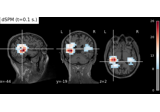Compute MNE-dSPM inverse solution on evoked data in volume source space

Compute MNE-dSPM inverse solution on evoked data in volume source space
bin(width, tstart=None, tstop=None, func=<function mean>)[source]#

Return a source estimate object with data summarized over time bins.

Time bins of `width` seconds. This method is intended for visualization only. No filter is applied to the data before binning, making the method inappropriate as a tool for downsampling data.

Parameters:
widthscalar

Width of the individual bins in seconds.

tstart

Time point where the first bin starts. The default is the first time point of the stc.

tstop

Last possible time point contained in a bin (if the last bin would be shorter than width it is dropped). The default is the last time point of the stc.

func`callable()`

Function that is applied to summarize the data. Needs to accept a numpy.array as first input and an `axis` keyword argument.

Returns:
stc

The binned source estimate.

copy()[source]#

Return copy of source estimate instance.

Returns:
stcinstance of `SourceEstimate`

A copy of the source estimate.

crop(tmin=None, tmax=None, include_tmax=True)[source]#

Restrict SourceEstimate to a time interval.

Parameters:
tmin

The first time point in seconds. If None the first present is used.

tmax

The last time point in seconds. If None the last present is used.

include_tmaxbool

If True (default), include tmax. If False, exclude tmax (similar to how Python indexing typically works).

New in v0.19.

Returns:
stcinstance of `SourceEstimate`

The cropped source estimate.

Examples using `crop`:Compute MNE-dSPM inverse solution on evoked data in volume source space

Compute MNE-dSPM inverse solution on evoked data in volume source space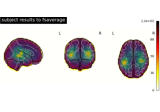Morph volumetric source estimate

Morph volumetric source estimate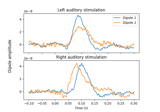Computing source timecourses with an XFit-like multi-dipole model

Computing source timecourses with an XFit-like multi-dipole model
property data#

Numpy array of source estimate data.

extract_label_time_course(labels, src, mode='auto', allow_empty=False, *, mri_resolution=True, verbose=None)[source]#

Extract label time courses for lists of labels.

This function will extract one time course for each label. The way the time courses are extracted depends on the mode parameter.

Parameters:
labels

If using a surface or mixed source space, this should be the `Label`’s for which to extract the time course. If working with whole-brain volume source estimates, this must be one of:

• a string path to a FreeSurfer atlas for the subject (e.g., their ‘aparc.a2009s+aseg.mgz’) to extract time courses for all volumes in the atlas

• a two-element list or tuple, the first element being a path to an atlas, and the second being a list or dict of `volume_labels` to extract (see `mne.setup_volume_source_space()` for details).

Changed in version 0.21.0: Support for volume source estimates.

srcinstance of `SourceSpaces`

The source spaces for the source time courses.

mode`str`

Extraction mode, see Notes.

allow_empty

`False` (default) will emit an error if there are labels that have no vertices in the source estimate. `True` and `'ignore'` will return all-zero time courses for labels that do not have any vertices in the source estimate, and True will emit a warning while and “ignore” will just log a message.

Changed in version 0.21.0: Support for “ignore”.

mri_resolutionbool

If True (default), the volume source space will be upsampled to the original MRI resolution via trilinear interpolation before the atlas values are extracted. This ensnures that each atlas label will contain source activations. When False, only the original source space points are used, and some atlas labels thus may not contain any source space vertices.

New in v0.21.0.

verbose

Control verbosity of the logging output. If `None`, use the default verbosity level. See the logging documentation and `mne.verbose()` for details. Should only be passed as a keyword argument.

Returns:
label_tc`array` | `list` (or generator) of `array`, shape (n_labels[, n_orient], n_times)

Extracted time course for each label and source estimate.

`extract_label_time_course`

Extract time courses for multiple STCs.

Notes

Valid values for `mode` are:

• `'max'`

Maximum absolute value across vertices at each time point within each label.

• `'mean'`

Average across vertices at each time point within each label. Ignores orientation of sources for standard source estimates, which varies across the cortical surface, which can lead to cancellation. Vector source estimates are always in XYZ / RAS orientation, and are thus already geometrically aligned.

• `'mean_flip'`

Finds the dominant direction of source space normal vector orientations within each label, applies a sign-flip to time series at vertices whose orientation is more than 90° different from the dominant direction, and then averages across vertices at each time point within each label.

• `'pca_flip'`

Applies singular value decomposition to the time courses within each label, and uses the first right-singular vector as the representative label time course. This signal is scaled so that its power matches the average (per-vertex) power within the label, and sign-flipped by multiplying by `np.sign(u @ flip)`, where `u` is the first left-singular vector and `flip` is the same sign-flip vector used when `mode='mean_flip'`. This sign-flip ensures that extracting time courses from the same label in similar STCs does not result in 180° direction/phase changes.

• `'auto'` (default)

Uses `'mean_flip'` when a standard source estimate is applied, and `'mean'` when a vector source estimate is supplied.

• `None`

No aggregation is performed, and an array of shape `(n_vertices, n_times)` is returned.

New in v0.21: Support for `'auto'`, vector, and volume source estimates.

The only modes that work for vector and volume source estimates are `'mean'`, `'max'`, and `'auto'`.

get_peak(tmin=None, tmax=None, mode='abs', vert_as_index=False, time_as_index=False)[source]#

Get location and latency of peak amplitude.

Parameters:
tmin

The minimum point in time to be considered for peak getting.

tmax

The maximum point in time to be considered for peak getting.

mode{‘pos’, ‘neg’, ‘abs’}

How to deal with the sign of the data. If ‘pos’ only positive values will be considered. If ‘neg’ only negative values will be considered. If ‘abs’ absolute values will be considered. Defaults to ‘abs’.

vert_as_indexbool

Whether to return the vertex index (True) instead of of its ID (False, default).

time_as_indexbool

Whether to return the time index (True) instead of the latency (False, default).

Returns:
pos`int`

The vertex exhibiting the maximum response, either ID or index.

latency`float`

The latency in seconds.

in_label(label, mri, src, *, verbose=None)[source]#

Get a source estimate object restricted to a label.

SourceEstimate contains the time course of activation of all sources inside the label.

Parameters:
label

The label to use. Can be the name of a label if using a standard FreeSurfer atlas, or an integer value to extract from the `mri`.

mri`str`

Path to the atlas to use.

srcinstance of `SourceSpaces`

The volumetric source space. It must be a single, whole-brain volume.

verbose

Control verbosity of the logging output. If `None`, use the default verbosity level. See the logging documentation and `mne.verbose()` for details. Should only be passed as a keyword argument.

Returns:
stc

The source estimate restricted to the given label.

Notes

New in v0.21.0.

mean()[source]#

Make a summary stc file with mean over time points.

Returns:
stc

The modified stc.

plot(src, subject=None, subjects_dir=None, mode='stat_map', bg_img='T1.mgz', colorbar=True, colormap='auto', clim='auto', transparent='auto', show=True, initial_time=None, initial_pos=None, verbose=None)[source]#

Plot Nutmeg style volumetric source estimates using nilearn.

Parameters:
srcinstance of `SourceSpaces` | instance of `SourceMorph`

The source space. Can also be a SourceMorph to morph the STC to a new subject (see Examples).

Changed in version 0.18: Support for `SpatialImage`.

subject

The FreeSurfer subject name. If `None`, `stc.subject` will be used.

subjects_dir

The path to the directory containing the FreeSurfer subjects reconstructions. If `None`, defaults to the `SUBJECTS_DIR` environment variable.

mode`'stat_map'` | `'glass_brain'`

The plotting mode to use. For `'glass_brain'`, activation absolute values are displayed after being transformed to a standard MNI brain.

bg_imginstance of `SpatialImage` | `str`

The background image used in the nilearn plotting function. Can also be a string to use the `bg_img` file in the subject’s MRI directory (default is `'T1.mgz'`). Not used in “glass brain” plotting.

colorbarbool, optional

If True, display a colorbar on the right of the plots.

colormap`str` | `np.ndarray` of `float`, shape(n_colors, 3 | 4)

Name of colormap to use or a custom look up table. If array, must be (n x 3) or (n x 4) array for with RGB or RGBA values between 0 and 255.

clim

Colorbar properties specification. If ‘auto’, set clim automatically based on data percentiles. If dict, should contain:

`kind`‘value’ | ‘percent’

Flag to specify type of limits.

`lims`list | np.ndarray | tuple of float, 3 elements

Lower, middle, and upper bounds for colormap.

`pos_lims`list | np.ndarray | tuple of float, 3 elements

Lower, middle, and upper bound for colormap. Positive values will be mirrored directly across zero during colormap construction to obtain negative control points.

Note

Only one of `lims` or `pos_lims` should be provided. Only sequential colormaps should be used with `lims`, and only divergent colormaps should be used with `pos_lims`.

transparent

If True: use a linear transparency between fmin and fmid and make values below fmin fully transparent (symmetrically for divergent colormaps). None will choose automatically based on colormap type.

showbool

Show figures if True. Defaults to True.

initial_time

The initial time to plot. Can be None (default) to use the time point with the maximal absolute value activation across all voxels or the `initial_pos` voxel (if `initial_pos is None` or not, respectively).

New in v0.19.

initial_pos`ndarray`, shape (3,) | `None`

The initial position to use (in m). Can be None (default) to use the voxel with the maximum absolute value activation across all time points or at `initial_time` (if `initial_time is None` or not, respectively).

New in v0.19.

verbose

Control verbosity of the logging output. If `None`, use the default verbosity level. See the logging documentation and `mne.verbose()` for details. Should only be passed as a keyword argument.

Returns:
figinstance of `Figure`

The figure.

Notes

Click on any of the anatomical slices to explore the time series. Clicking on any time point will bring up the corresponding anatomical map.

The left and right arrow keys can be used to navigate in time. To move in time by larger steps, use shift+left and shift+right.

In `'glass_brain'` mode, values are transformed to the standard MNI brain using the FreeSurfer Talairach transformation `\$SUBJECTS_DIR/\$SUBJECT/mri/transforms/talairach.xfm`.

New in v0.17.

Changed in version 0.19: MRI volumes are automatically transformed to MNI space in `'glass_brain'` mode.

Examples

Passing a `mne.SourceMorph` as the `src` parameter can be useful for plotting in a different subject’s space (here, a `'sample'` STC in `'fsaverage'`’s space):

```>>> morph = mne.compute_source_morph(src_sample, subject_to='fsaverage')
>>> fig = stc_vol_sample.plot(morph)
```

Examples using `plot`: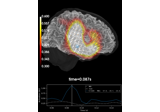Source reconstruction using an LCMV beamformer

Source reconstruction using an LCMV beamformer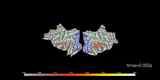Visualize source time courses (stcs)

Visualize source time courses (stcs)
plot_3d(subject=None, surface='white', hemi='both', colormap='auto', time_label='auto', smoothing_steps=10, transparent=True, alpha=0.1, time_viewer='auto', subjects_dir=None, figure=None, views='axial', colorbar=True, clim='auto', cortex='classic', size=800, background='black', foreground=None, initial_time=None, time_unit='s', backend='auto', spacing='oct6', title=None, show_traces='auto', src=None, volume_options=1.0, view_layout='vertical', add_data_kwargs=None, brain_kwargs=None, verbose=None)[source]#

Plot SourceEstimate.

Parameters:
subject

The FreeSurfer subject name. If `None`, `stc.subject` will be used.

surface`str`

The type of surface (inflated, white etc.).

hemi`str`

Hemisphere id (ie `'lh'`, `'rh'`, `'both'`, or `'split'`). In the case of `'both'`, both hemispheres are shown in the same window. In the case of `'split'` hemispheres are displayed side-by-side in different viewing panes.

colormap`str` | `np.ndarray` of `float`, shape(n_colors, 3 | 4)

Name of colormap to use or a custom look up table. If array, must be (n x 3) or (n x 4) array for with RGB or RGBA values between 0 and 255. The default (‘auto’) uses `'hot'` for one-sided data and ‘mne’ for two-sided data.

time_label

Format of the time label (a format string, a function that maps floating point time values to strings, or None for no label). The default is `'auto'`, which will use `time=%0.2f ms` if there is more than one time point.

smoothing_steps`int`

The amount of smoothing.

transparent

If True: use a linear transparency between fmin and fmid and make values below fmin fully transparent (symmetrically for divergent colormaps). None will choose automatically based on colormap type.

alpha`float`

Alpha value to apply globally to the overlay. Has no effect with mpl backend.

time_viewer

Display time viewer GUI. Can also be ‘auto’, which will mean True for the PyVista backend and False otherwise.

Changed in version 0.20.0: “auto” mode added.

subjects_dir

The path to the directory containing the FreeSurfer subjects reconstructions. If `None`, defaults to the `SUBJECTS_DIR` environment variable.

figureinstance of `Figure3D` | instance of `matplotlib.figure.Figure` | `list` | `int` | `None`

If None, a new figure will be created. If multiple views or a split view is requested, this must be a list of the appropriate length. If int is provided it will be used to identify the PyVista figure by it’s id or create a new figure with the given id. If an instance of matplotlib figure, mpl backend is used for plotting.

views

View to use. Using multiple views (list) is not supported for mpl backend. See `Brain.show_view` for valid string options.

When plotting a standard SourceEstimate (not volume, mixed, or vector) and using the PyVista backend, `views='flat'` is also supported to plot cortex as a flatmap.

Using multiple views (list) is not supported by the matplotlib backend.

Changed in version 0.21.0: Support for flatmaps.

colorbarbool

If True, display colorbar on scene.

clim

Colorbar properties specification. If ‘auto’, set clim automatically based on data percentiles. If dict, should contain:

`kind`‘value’ | ‘percent’

Flag to specify type of limits.

`lims`list | np.ndarray | tuple of float, 3 elements

Lower, middle, and upper bounds for colormap.

`pos_lims`list | np.ndarray | tuple of float, 3 elements

Lower, middle, and upper bound for colormap. Positive values will be mirrored directly across zero during colormap construction to obtain negative control points.

Note

Only one of `lims` or `pos_lims` should be provided. Only sequential colormaps should be used with `lims`, and only divergent colormaps should be used with `pos_lims`.

cortex

Specifies how binarized curvature values are rendered. Either the name of a preset Brain cortex colorscheme (one of `'classic'`, `'bone'`, `'low_contrast'`, or `'high_contrast'`), or the name of a colormap, or a tuple with values `(colormap, min, max, reverse)` to fully specify the curvature colors. Has no effect with the matplotlib backend.

size

The size of the window, in pixels. can be one number to specify a square window, or the (width, height) of a rectangular window. Has no effect with mpl backend.

backgroundmatplotlib color

Color of the background of the display window.

foregroundmatplotlib color | `None`

Color of the foreground of the display window. Has no effect with mpl backend. None will choose white or black based on the background color.

initial_time

The time to display on the plot initially. `None` to display the first time sample (default).

time_unit`'s'` | `'ms'`

Whether time is represented in seconds (“s”, default) or milliseconds (“ms”).

backend`'auto'` | `'pyvistaqt'` | `'matplotlib'`

Which backend to use. If `'auto'` (default), tries to plot with pyvistaqt, but resorts to matplotlib if no 3d backend is available.

New in v0.15.0.

spacing`str`

Only affects the matplotlib backend. The spacing to use for the source space. Can be `'ico#'` for a recursively subdivided icosahedron, `'oct#'` for a recursively subdivided octahedron, or `'all'` for all points. In general, you can speed up the plotting by selecting a sparser source space. Defaults to ‘oct6’.

New in v0.15.0.

title

Title for the figure. If None, the subject name will be used.

New in v0.17.0.

show_traces

If True, enable interactive picking of a point on the surface of the brain and plot its time course. This feature is only available with the PyVista 3d backend, and requires `time_viewer=True`. Defaults to ‘auto’, which will use True if and only if `time_viewer=True`, the backend is PyVista, and there is more than one time point. If float (between zero and one), it specifies what proportion of the total window should be devoted to traces (True is equivalent to 0.25, i.e., it will occupy the bottom 1/4 of the figure).

New in v0.20.0.

srcinstance of `SourceSpaces` | `None`

The source space corresponding to the source estimate. Only necessary if the STC is a volume or mixed source estimate.

volume_options

Options for volumetric source estimate plotting, with key/value pairs:

• `'resolution'`float | None

Resolution (in mm) of volume rendering. Smaller (e.g., 1.) looks better at the cost of speed. None (default) uses the volume source space resolution, which is often something like 7 or 5 mm, without resampling.

• `'blending'`str

Can be “mip” (default) for maximum intensity projection or “composite” for composite blending using alpha values.

• `'alpha'`float | None

Alpha for the volumetric rendering. Defaults are 0.4 for vector source estimates and 1.0 for scalar source estimates.

• `'surface_alpha'`float | None

Alpha for the surface enclosing the volume(s). None (default) will use half the volume alpha. Set to zero to avoid plotting the surface.

• `'silhouette_alpha'`float | None

Alpha for a silhouette along the outside of the volume. None (default) will use `0.25 * surface_alpha`.

• `'silhouette_linewidth'`float

The line width to use for the silhouette. Default is 2.

A float input (default 1.) or None will be used for the `'resolution'` entry.

view_layout`str`

Can be “vertical” (default) or “horizontal”. When using “horizontal” mode, the PyVista backend must be used and hemi cannot be “split”.

Additional arguments to brain.add_data (e.g., `dict(time_label_size=10)`).

brain_kwargs

Additional arguments to the `mne.viz.Brain` constructor (e.g., `dict(silhouette=True)`).

verbose

Control verbosity of the logging output. If `None`, use the default verbosity level. See the logging documentation and `mne.verbose()` for details. Should only be passed as a keyword argument.

Returns:
figure

An instance of `mne.viz.Brain` or matplotlib figure.

Notes

Flatmaps are available by default for `fsaverage` but not for other subjects reconstructed by FreeSurfer. We recommend using `mne.compute_source_morph()` to morph source estimates to `fsaverage` for flatmap plotting. If you want to construct your own flatmap for a given subject, these links might help:

Examples using `plot_3d`: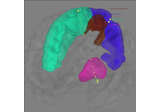Working with sEEG data

Working with sEEG data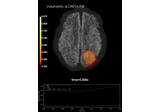Plot point-spread functions (PSFs) for a volume

Plot point-spread functions (PSFs) for a volume

Resample data.

If appropriate, an anti-aliasing filter is applied before resampling. See Resampling and decimating data for more information.

Parameters:
sfreq`float`

New sample rate to use.

Amount to pad the start and end of the data. Can also be “auto” to use a padding that will result in a power-of-two size (can be much faster).

window

Window to use in resampling. See `scipy.signal.resample()`.

n_jobs

The number of jobs to run in parallel. If `-1`, it is set to the number of CPU cores. Requires the `joblib` package. `None` (default) is a marker for ‘unset’ that will be interpreted as `n_jobs=1` (sequential execution) unless the call is performed under a `joblib.parallel_config` context manager that sets another value for `n_jobs`.

verbose

Control verbosity of the logging output. If `None`, use the default verbosity level. See the logging documentation and `mne.verbose()` for details. Should only be passed as a keyword argument.

Returns:
stcinstance of `SourceEstimate`

The resampled source estimate.

Notes

For some data, it may be more accurate to use npad=0 to reduce artifacts. This is dataset dependent – check your data!

Note that the sample rate of the original data is inferred from tstep.

save(fname, ftype='stc', *, overwrite=False, verbose=None)[source]#

Save the source estimates to a file.

Parameters:
fnamepath-like

The stem of the file name. The stem is extended with `"-vl.stc"` or `"-vl.w"`.

ftype`str`

File format to use. Allowed values are `"stc"` (default), `"w"`, and `"h5"`. The `"w"` format only supports a single time point.

overwritebool

If True (default False), overwrite the destination file if it exists.

New in v1.0.

verbose

Control verbosity of the logging output. If `None`, use the default verbosity level. See the logging documentation and `mne.verbose()` for details. Should only be passed as a keyword argument.

save_as_volume(fname, src, dest='mri', mri_resolution=False, format='nifti1', *, overwrite=False, verbose=None)[source]#

Save a volume source estimate in a NIfTI file.

Parameters:
fnamepath-like

The name of the generated nifti file.

src`list`

The list of source spaces (should all be of type volume).

dest`'mri'` | `'surf'`

If `'mri'` the volume is defined in the coordinate system of the original T1 image. If `'surf'` the coordinate system of the FreeSurfer surface is used (Surface RAS).

mri_resolutionbool

It True the image is saved in MRI resolution.

format`str`

Either `'nifti1'` (default) or `'nifti2'`.

New in v0.17.

overwritebool

If True (default False), overwrite the destination file if it exists.

New in v1.0.

verbose

Control verbosity of the logging output. If `None`, use the default verbosity level. See the logging documentation and `mne.verbose()` for details. Should only be passed as a keyword argument.

New in v1.0.

Returns:
imginstance `Nifti1Image`

The image object.

Notes

New in v0.9.0.

property sfreq#

Sample rate of the data.

property shape#

Shape of the data.

sqrt()[source]#

Take the square root.

Returns:
stcinstance of `SourceEstimate`

A copy of the SourceEstimate with sqrt(data).

sum()[source]#

Make a summary stc file with sum over time points.

Returns:
stc

The modified stc.

time_as_index(times, use_rounding=False)[source]#

Convert time to indices.

Parameters:
timeslist-like | `float` | `int`

List of numbers or a number representing points in time.

use_roundingbool

If True, use rounding (instead of truncation) when converting times to indices. This can help avoid non-unique indices.

Returns:
index`ndarray`

Indices corresponding to the times supplied.

property times#

A timestamp for each sample.

property tmin#

The first timestamp.

to_data_frame(index=None, scalings=None, long_format=False, time_format=None, *, verbose=None)[source]#

Export data in tabular structure as a pandas DataFrame.

Vertices are converted to columns in the DataFrame. By default, an additional column “time” is added, unless `index='time'` (in which case time values form the DataFrame’s index).

Parameters:
index‘time’ | `None`

Kind of index to use for the DataFrame. If `None`, a sequential integer index (`pandas.RangeIndex`) will be used. If `'time'`, a `pandas.Index` or `pandas.TimedeltaIndex` will be used (depending on the value of `time_format`). Defaults to `None`.

scalings

Scaling factor applied to the channels picked. If `None`, defaults to `dict(eeg=1e6, mag=1e15, grad=1e13)` — i.e., converts EEG to µV, magnetometers to fT, and gradiometers to fT/cm.

long_formatbool

If True, the DataFrame is returned in long format where each row is one observation of the signal at a unique combination of time point and vertex. Defaults to `False`.

time_format

Desired time format. If `None`, no conversion is applied, and time values remain as float values in seconds. If `'ms'`, time values will be rounded to the nearest millisecond and converted to integers. If `'timedelta'`, time values will be converted to `pandas.Timedelta` values. Default is `None`.

New in v0.20.

verbose

Control verbosity of the logging output. If `None`, use the default verbosity level. See the logging documentation and `mne.verbose()` for details. Should only be passed as a keyword argument.

Returns:
dfinstance of `pandas.DataFrame`

A dataframe suitable for usage with other statistical/plotting/analysis packages.

transform(func, idx=None, tmin=None, tmax=None, copy=False)[source]#

Apply linear transform.

The transform is applied to each source time course independently.

Parameters:
func`callable()`

The transform to be applied, including parameters (see, e.g., `functools.partial()`). The first parameter of the function is the input data. The first two dimensions of the transformed data should be (i) vertices and (ii) time. See Notes for details.

idx

Indices of source time courses for which to compute transform. If None, all time courses are used.

tmin

First time point to include (ms). If None, self.tmin is used.

tmax

Last time point to include (ms). If None, self.tmax is used.

copybool

If True, return a new instance of SourceEstimate instead of modifying the input inplace.

Returns:
stcs

The transformed stc or, in the case of transforms which yield N-dimensional output (where N > 2), a list of stcs. For a list, copy must be True.

Notes

Transforms which yield 3D output (e.g. time-frequency transforms) are valid, so long as the first two dimensions are vertices and time. In this case, the copy parameter must be True and a list of SourceEstimates, rather than a single instance of SourceEstimate, will be returned, one for each index of the 3rd dimension of the transformed data. In the case of transforms yielding 2D output (e.g. filtering), the user has the option of modifying the input inplace (copy = False) or returning a new instance of SourceEstimate (copy = True) with the transformed data.

Applying transforms can be significantly faster if the SourceEstimate object was created using “(kernel, sens_data)”, for the “data” parameter as the transform is applied in sensor space. Inverse methods, e.g., “apply_inverse_epochs”, or “apply_lcmv_epochs” do this automatically (if possible).

transform_data(func, idx=None, tmin_idx=None, tmax_idx=None)[source]#

Get data after a linear (time) transform has been applied.

The transform is applied to each source time course independently.

Parameters:
func`callable()`

The transform to be applied, including parameters (see, e.g., `functools.partial()`). The first parameter of the function is the input data. The first return value is the transformed data, remaining outputs are ignored. The first dimension of the transformed data has to be the same as the first dimension of the input data.

idx

Indicices of source time courses for which to compute transform. If None, all time courses are used.

tmin_idx

Index of first time point to include. If None, the index of the first time point is used.

tmax_idx

Index of the first time point not to include. If None, time points up to (and including) the last time point are included.

Returns:
data_t`ndarray`

The transformed data.

Notes

Applying transforms can be significantly faster if the SourceEstimate object was created using “(kernel, sens_data)”, for the “data” parameter as the transform is applied in sensor space. Inverse methods, e.g., “apply_inverse_epochs”, or “apply_lcmv_epochs” do this automatically (if possible).

property tstep#

The change in time between two consecutive samples (1 / sfreq).

## Examples using `mne.VolSourceEstimate`#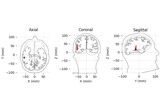Source localization with equivalent current dipole (ECD) fit

Source localization with equivalent current dipole (ECD) fitSource reconstruction using an LCMV beamformer

Source reconstruction using an LCMV beamformerVisualize source time courses (stcs)

Visualize source time courses (stcs)Working with sEEG data

Working with sEEG dataCompute MNE-dSPM inverse solution on evoked data in volume source space

Compute MNE-dSPM inverse solution on evoked data in volume source spaceMorph volumetric source estimate

Morph volumetric source estimateComputing source timecourses with an XFit-like multi-dipole model

Computing source timecourses with an XFit-like multi-dipole modelPlot point-spread functions (PSFs) for a volume

Plot point-spread functions (PSFs) for a volume